Next: 2.2 Modified Nodal Approach Up: 2. Circuit Equations Previous: 2. Circuit Equations

# 2.1 Nodal Approach

For the derivation of the nodal approach (NA) a circuit containing linear conductances and independent current sources is assumed. The direction of the current flow is defined in such a way that for both passive elements and sources the current-voltage product is positive, that is P = V . I > 0 and P = V . J > 0 (see Fig. 2.1).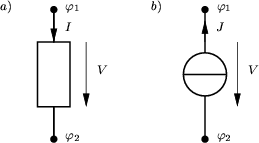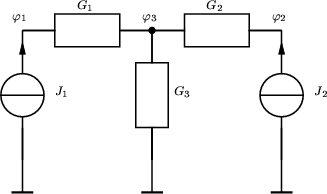The independent variables of the NA are the node voltages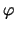of each circuit node to a reference node which can be chosen arbitrarily. This choice guarantees that KVL is fulfilled which requires that the sum of all voltages around any loop in the circuit are zero. Considering an arbitrary loop in an arbitrary circuit the sum of the voltages can be written as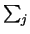Vj = (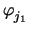-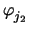) + (-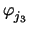) +...+ (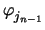-) = 0 (2.1)

Kirchhoff's current law (KCL) is applied to each node other than the reference node in the circuit such that the summation of the currents leaving the node is equal to zero. This procedure is best illustrated on the example circuit shown in Fig. 2.2. Summing the currents at all nodes one gets

 node 0 : G3 . (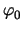-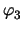) = - J1 - J2 (2.2) node 1 : G1 . (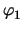-) = J1 (2.3) node 2 : G2 . (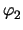-) = J2 (2.4) node 3 : G1 . (-) + G2 . (-) + G3 . (-) = 0 (2.5)

or in matrix and vector notation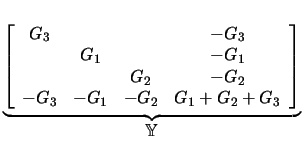.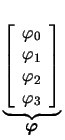=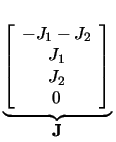. (2.6)

The matrix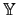is the admittance matrix of the circuit. However, it is obvious that the equations (2.2) - (2.5) are not independent. In general it can be shown that for a circuit containing N nodes, exactly N - 1 independent KCL equations can be formulated . As the reference voltage is fixed anyway, there are N - 1 equations for the remaining N - 1 unknowns. The absolute value of the reference voltage is of course arbitrary and normally assumed to be zero.

Another important issue is thatcan be assembled by inspection on a per-element basis. The admittance matrices of the devices can simply be superpositioned to yield the circuit admittance matrix. Current sources contribute to the current source vector J. Therefore these contributions are commonly refered to as stamps as they can be directly stamped into the system matrix element per element without the need to consider the rest of the circuit.G =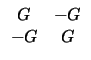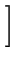(2.7)

whereas the contribution of an ideal current source J with the current J0 to the current source vector is given as

 JJ =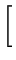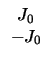(2.8)

The stamps normally contain contributions to bothand J, hence a stamp for a current source with shunt conductance reads
 yx, y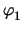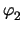J n1 G - G J0 n2 - G G - J0
and is simply a superposition of a conductor and a current source.

Non-linear branch relations are handled by introducing linearized companion models. A general non-linear device with branch relation

 I = I() (2.9)

can be linearized using a Taylor expansion around the last iteration k which gives

 I(k + 1) =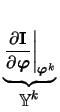.k + 1 +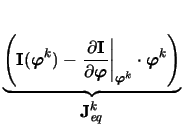(2.10) Jeqk = I(k) -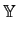k .k   . (2.11)

The partial derivative in the first term is the linearized admittance matrixk while the second term Jeqk is a constant current source which is independent of the new solutionk + 1. Hence, at each iteration each non-linear device can be replaced by linear conductances and current sources resulting in a Newton-like method. Considering the branch relation of a diode

 ID =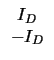(2.12) with ID = IS .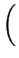exp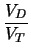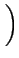- 1(2.13)

one gets the following stamp
 yx, yJ n1 gmk - gmk Jeqk n2 - gmk gmk - Jeqk
with

 gmk =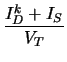(2.14) Jeqk = IDk - gm . VDk (2.15)

For circuits containing only conductances and current sources, the condition of the resulting equation system is very good. In this case the NA produces diagonal-dominant matrices which are well suited for iterative solution procedures. Two additional devices can be modeled using the NA, namely a voltage controlled current source (cf. Section 2.3.3.8) and the gyrator (cf. Section 2.3.3.12). These devices destroy the diagonal dominance of the circuit admittance matrix.

One disadvantage of the NA is the inadequate treatment of voltage sources. Ideal voltage sources and current controlled elements cannot be modeled with this approach. However, a very large class of integrated circuits can be accommodated by adding a provision for grounded sources. Other workarounds for floating voltage sources have been proposed which can be quite awkward to handle [53,65]. To model current dependencies small or negative resistances have been introduced which result in numerical instabilities .Next: 2.2 Modified Nodal Approach Up: 2. Circuit Equations Previous: 2. Circuit Equations
Tibor Grasser
1999-05-31